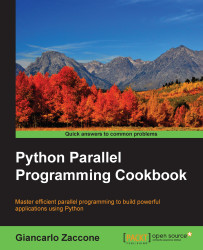•#### Python Parallel Programming Cookbook#### Overview of this book

This book will teach you parallel programming techniques using examples in Python and will help you explore the many ways in which you can write code that allows more than one process to happen at once. Starting with introducing you to the world of parallel computing, it moves on to cover the fundamentals in Python. This is followed by exploring the thread-based parallelism model using the Python threading module by synchronizing threads and using locks, mutex, semaphores queues, GIL, and the thread pool. Next you will be taught about process-based parallelism where you will synchronize processes using message passing along with learning about the performance of MPI Python Modules. You will then go on to learn the asynchronous parallel programming model using the Python asyncio module along with handling exceptions. Moving on, you will discover distributed computing with Python, and learn how to install a broker, use Celery Python Module, and create a worker. You will understand anche Pycsp, the Scoop framework, and disk modules in Python. Further on, you will learnGPU programming withPython using the PyCUDA module along with evaluating performance limitations.
Python Parallel Programming CookbookCreditswww.PacktPub.comPrefaceFree Chapter
Getting Started with Parallel Computing and PythonProcess-based ParallelismAsynchronous ProgrammingDistributed PythonGPU Programming with PythonIndex## Using GPU-accelerated libraries with NumbaPro

NumbaPro provides a Python wrap for CUDA libraries for numerical computing. Each code using these libraries will get a significant speedup without writing any GPU-specific code. The libraries are explained as follows:

• cuBLAS: This is a library developed by NVIDIA that provides the main functions of linear algebra to run on a GPU. Like the Basic Linear Algebra Subprograms (BLAS) library that implements the functions of linear algebra on the CPU, the cuBLAS library classifies its functions into three levels:

• Level 1: Vector operations

• Level 2: Transactions between a matrix and vector

• Level 3: Operations between matrices

The division of these functions in the three levels is based on the number of nested loops that are needed to perform the selected operation. More precisely, the operations of the level are essential cycles that are geared to complete the execution of the selected function.

• cuFFT: This provides a simple interface to calculate the...